# AP 8th Maths Mock Test 2023 For Chapter – 1 : “Rational Numbers” Online Practice Test

1. How is -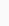is expressed as a rational number with denominator 20 ?
2. Expressandwith same denominator.
3. Multiplicative inverse of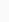is
4. Multiplicative inverse ofis
5. Multiplicative inverse of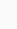is
6. Multiplicative identity in the set of rationals is _____
7. The reciprocal of '0' is _____
8. The reciprocal of '0' is _____
9. The reciprocal of '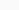' is _____
10. What is the sum of additive inverse and multiplicative inverse of -1?
11. What is the sum of additive inverse and multiplicative inverse of 1?
12.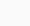can be expressed as a rational number as _______
13. What is the multiplicative inverse of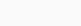?
14. Which of the following is correct ?
15. Which of the following are 3 rational numbers between -2 and -1 ?
16. Which of the following are 3 rational numbers between -3 and -2 ?
17. Which of the following are 3 rational numbers between 3 and 4 ?
18. Which of the following are 3 rational numbers between 4 and 7 ?
19. A rational number between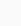andis _____
20. A rational number betweenandis _____
21. A rational number betweenandis _____
22.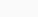= _____
23.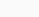= _____
24. If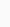is the fractional form ofthen p+q = ____
25. Ifis the fractional form of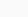then p+q = ____
26. Ifis the fractional form ofthen p+q = ____
27. If x =, y =, then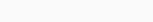= ______
28. The denominator of a fraction which equals to the decimal fraction of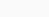is _____
29.+9.1 = _____
30. The reciprocal of 9 lies in the number system _____
31. The differences of the period and periodicity ofis ______
32. The product of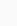and reciprocal ofis _____
33. The sum of 2 rational numbers is 8 and one of them is. Then the second number is ____
34. The negative of -2 multiplied by its reciprocal gives
35. Which of the following rational has a terminating decimal ?
36. ax - y.ay - z.az - x = ..............
37. Which of the rational numbers -, -, -, -is the greatest ?
38. The reciprocal of 0.75 is ................
39.-=
40. If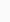=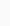then x = ..............
41. Which of the following is not a rational number ?
42. Rama :is a rational number and 5 is only a natural number
Shyama : Bothand 5 are rational numbers
Which of the statements are true ?
43. Assertion A : Set of integers is closed under subtraction
Reason R : Difference of two integers is not necessarily an integer
Which of the following statements are true ?
44. a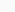b = ba holds only if ..............
45. Which of the following set of numbers has no identity element under + ?
46. Which of the following is different among  the following rationals ?
47. 0.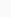+ 0.+ 0.= .............
48.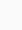=
49. In the following , multiplicative associative property is
50.= ................
51. Any integer division by zero =
52. The rational number that does not have any reciprocal is ..............
53. Which of the following is only an integer but not a rational number ?
54. Find the natural number but not a rational number ?
55. The set of integers is not closed under
56.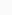a , b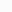Q we have a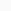bQ . This states ...................... property under multiplication of rational number
57.a , bQ we have a  + b = b + a . This states ...................... property under addition in Q
58. Commutative property does not hold under subtraction in the set of ...................
59.aQ we have a1 = a =  1a . This indicates ...................... property
60. Additive identity in the set of rational number is ..............
61. Which property can be used in computing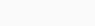+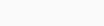?
62. If 'n' is a prime number , then  the periodicity ofis ......................
63. How many integers are there in between -1 and 1 ?
64. The set of rationals denoted by the letter ..............
65. IF (x + y)z = 1 then z is a multiplicative inverse of ..................
66. Write Y if Yes and N if No in place of i , ii , iii , iv in the following table to complete it in order67. Match the following of Column - 1 with Column -2.

Column - 1                                                                   Column - 2
1.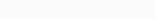(  )  p) Commutative property
2.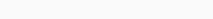(  )  q) Associative property
3.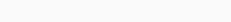(  )  r) Closure property
68. A bus is moving at an average speed of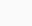km/hr. How much distance it will cover inhours ?
69. What is the multiplicative inverse of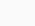?
70. The area of a rectangular park whose length ism and breadth is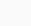m _____
71. Arrange the steps in order to express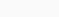inform
1. 10x = 157.3232.......
2. 1000x = 15732.3232........
3. Subtracting we get x =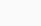4. Let x =72. Identify the rational number shown A marked in the following number line.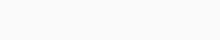73. Write a rational number for the points labelled with letters P, Q, R, S in order on number line.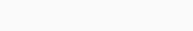74. Which letter of the number indicatesin the following ?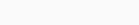75. The sets of natural numbers, whole numbers, integers and rational numbers can be put as in the following figures.
76. Integers are combination of
78. Identify the property in 2+3 = 3+2
79. Identify the property in 30 = 03
80. Identify the property in 3-2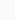2-3
81. Identify the property in 3223
82. Identify the property in 2+(3+0) = (2+3)+0
83. Identify the property in 2-(3-0)(2-3)-0
84. Identify the property in 2(30)(23)0
85. Identify the property in 2(30)(23)0
86. Do natural numbers obey associative property under addition ?
87. Do whole numbers obey associative property under addition ?
88. Do integers obey associative property under addition ?
89. Do integers obey associative property under addition ?
90. Do rational numbers obey associative property under addition ?
91. Do rational numbers obey associative property under multiplication ?
92. Do integers obey associative property under multiplication ?
93. Do whole numbers obey associative property under multiplication ?
94. Do natural numbers obey associative property under multiplication ?
95. {1 , 2 , 3 ,.........} is the set of
96. {0 , 1 , 2 , 3 ,.........} is the set of
97. {...........- 3 , - 2 , - 1 , 0 , 1 , 2 , 3 ,.........} is the set of
98. Rational numbers are ................. under addition
99. Hamid :is rational and 5 is natural number
Hamida :, 5 are rational
The correct statements are
100. Zero is